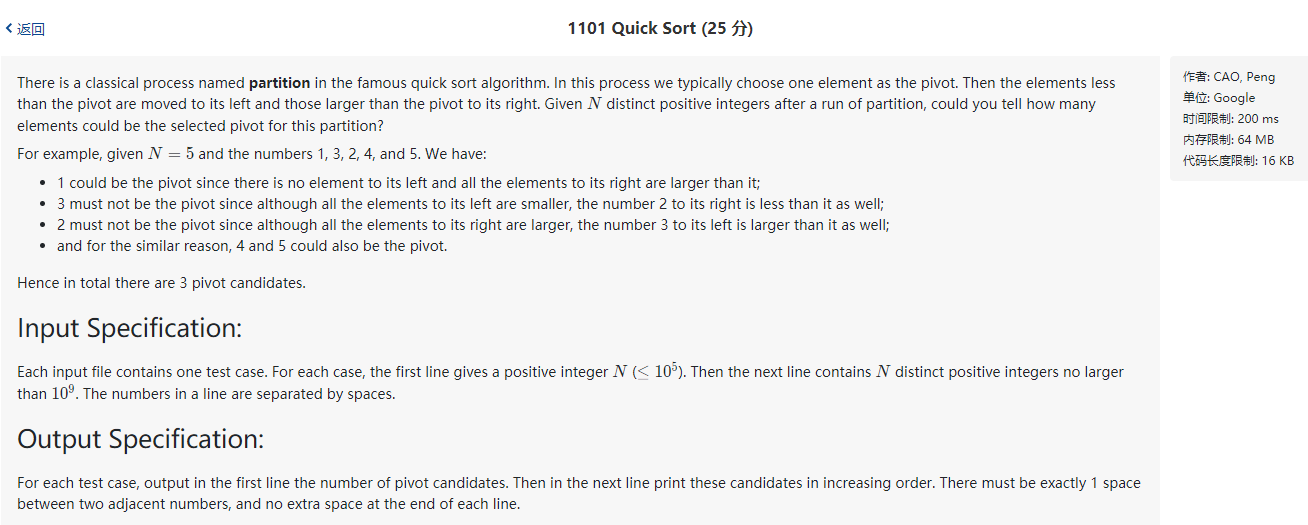``````PAT甲级1101.
``````

# A1101

## 题目## 样例

``````5
1 3 2 4 5
``````

``````3
1 4 5
``````

## 思路和坑点

根据主元的特点，在排好序的所在位置上，且左侧的数都小于自身，右侧的数都大于自身，由于题目中的数字都不同，因此只需要验证一侧即可。排序后，遍历数组一一验证是否满足主元的特征。 　　坑点
最后要多加一个换行符，估计是0个的情况下，主元不用输出但是仍要占据一行。（这个真是谜之判定）

## AC代码

``````#include<iostream>
#include<stdlib.h>
#include<stdio.h>
#include<string.h>
#include<math.h>
#include<algorithm>
#include<vector>
#include<string>
#include<map>
#include<unordered_map>
#include<queue>
#include<set>
#include<stack>
using namespace std;
//快速排序的特性，最终所选的主元都在自己应该在的位置上
//并且保证左边的都小于主元，右边的都大于主元
int main(void){
#ifdef ONLINE_JUDGE
#else
freopen("1.txt","r",stdin);
#endif
int n;
vector<int> v,s,out;
scanf("%d",&n);
v.resize(n); s.resize(n);
for(int i=0;i<n;i++){           //读入数据
scanf("%d",&v[i]);
s[i]=v[i];
}
int max=-1;                     //记录左侧的最大值
sort(s.begin(),s.end());        //对其中一个数组进行排序
for(int i=0;i<n;i++){
if(v[i]==s[i]&&v[i]>max)    //如果v中的一个元素在自己的排序后的位置上，且左边的数都小于自身
out.push_back(s[i]);    //则为主元，因为题中所有的数均不相同，因此一定满足右边的数都大于自身
max=max>v[i]?max:v[i];      //更新左侧最大值
}
cout<<out.size()<<'\n';         //输出结果
for(int i=0;i<out.size();i++){
if(i) putchar(' ');
printf("%d",out[i]);
}
putchar('\n');                  //谜之换行符，没有这一句，有一个测试点会格式错误
return 0;
}
``````